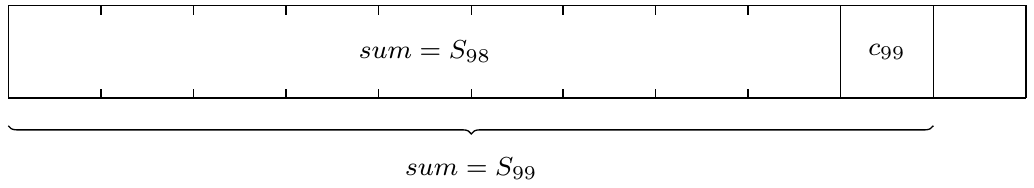#### Demystify Modular Arithmetic

Yesterday's daily problem is a puzzle (# 3926). The first question we should ask is why this puzzle is a math problem?

Because it is an excellent example of modular arithmetic's application. Modular arithmetic is an important number theory subject.

Surprising or not, no matter whether there are $2$ or $100$ known colors, at least $99$ prisoners can be saved. In fact, even if hats have thousands of different colors, the answer remains the same.

The tool to solve this problem is modular arithmetic operation. Many students feel modular arithmetic mysterious. However, the vast majority of its basic operations are the same as regular ones. For example, given integers $a$, $b$, $c$, and $M$, we have

$$a\pm b=c\implies a\pm b\equiv c\pmod{M}$$

and $$a+b\equiv c\pmod{M}\ \Leftrightarrow\ a \equiv c-b\pmod{M}$$

This puzzle can be solved by just using these basics. Let's assume hats have $2017$ different possible colors. We can soon see that the number of colors is irrelevant.

Firstly, the $100^{th}$ prisoner has absolutely no information available to him. Hence, his fate is in the God's hand. However, he will be able to help save everyone else. In order to do this, let's represent the given $2017$ colors using number $0$, $1$, $\cdots$, $2016$. Then, all the $100^{th}$ prisoner needs to do is to call out the color that is corresponding to the sum of the corresponding numbers of all the previous $99$ hats' colors. Let's call this sum as $S_{99}$.

Now, let's consider the $99^{th}$ prisoner. He knows $S_{99}$, and can compute $S_{98}$ which is the sum of the numbers in front of him. Then his color $c_{99}$ can be computed by $$c_{99}=S_{99}-S_{98}\pmod{2017}$$Now, let's move to the $98^{th}$ prisoner. Because all the terms but $c_{98}$ in the following equation are known, he can figure out $c_{98}$ as well. $$S_{97} + c_{98} + c_{99} = S_{99}\pmod{2017}$$

$$\therefore\ \ c_{98} = S_{99}-S_{97}-c_{99}\pmod{2017}$$

This process can continue till the first person in the file.

Modular arithmetic is tested in almost all math competitions at all levels. The book covers all aspects of this subject needed for middle and high school level contests.